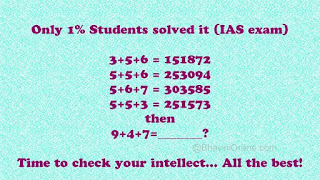## Saturday, 11 April 2020

### SOLUTION OF THIS PUZZLE

I came across this tough maths puzzle on Whatsapp today.
Many developing countries, especially those most threatened by climate changes, have also pushed for legal force and the wider international community has also promised itself that 2015 is the deadline for a legal pact. In the 2011 agreement in Durban, which began the process recently concluded in Paris, nations agreed to “develop a protocol, another legal instrument or an agreed outcome with legal force” no later than the end of 2015. But the Obama administration, in its capacity as a regent of the United States, does not want a legally binding treaty to emerge from the conference. That’s because, in truth, not everyone in the United States actually wants a deal.

### Answer to the Maths Puzzle:## SOLUTION OF THIS PUZZLE

The solution to the first 4 digits is pretty straight forward, and I am sure most of you must have easily got it.

It works out to be a+b+c = (a*b)(a*c)??

The last 2 digits are really tough to crack…

Here’s how you do it. Reverse of {(a×b)+(a×c)-c}

So the number would (a×b),(a×c),{(a×b)+(a×c)-c}reverse.

So for 1st one: 3+5+6=151872;
axb = 3×5 = 15; axc = 3×6 = 18;
{(a×b)+(a×c)-c} = ((3×5)+(3×6)-6} = 27
— Reverse of 27 is 72

So 9+4+7=_______?

axb = 9×4 = 36;
axc = 9×7 = 63;
{(a×b)+(a×c)-c} = ((9×4)+(9×7)-7} = (36+63-7)=92
— Reverse of 92 is 29.

Answer :::: Hence 9+4+7 = 366329